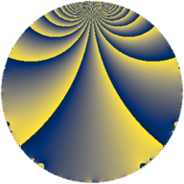# Properties

 Label 81.2.eLevel $81$ Weight $2$ Character orbit 81.e Rep. character $\chi_{81}(10,\cdot)$ Character field $\Q(\zeta_{9})$ Dimension $12$ Newform subspaces $1$ Sturm bound $18$ Trace bound $0$

# Related objects

## Defining parameters

 Level: $$N$$ $$=$$ $$81 = 3^{4}$$ Weight: $$k$$ $$=$$ $$2$$ Character orbit: $$[\chi]$$ $$=$$ 81.e (of order $$9$$ and degree $$6$$) Character conductor: $$\operatorname{cond}(\chi)$$ $$=$$ $$27$$ Character field: $$\Q(\zeta_{9})$$ Newform subspaces: $$1$$ Sturm bound: $$18$$ Trace bound: $$0$$

## Dimensions

The following table gives the dimensions of various subspaces of $$M_{2}(81, [\chi])$$.

Total New Old
Modular forms 72 24 48
Cusp forms 36 12 24
Eisenstein series 36 12 24

## Trace form

 $$12 q + 6 q^{2} - 6 q^{4} + 3 q^{5} - 6 q^{7} - 6 q^{8} + O(q^{10})$$ $$12 q + 6 q^{2} - 6 q^{4} + 3 q^{5} - 6 q^{7} - 6 q^{8} - 3 q^{10} - 3 q^{11} - 6 q^{13} - 15 q^{14} - 9 q^{17} - 3 q^{19} + 3 q^{20} + 3 q^{22} + 12 q^{23} + 3 q^{25} + 30 q^{26} - 12 q^{28} + 6 q^{29} + 3 q^{31} + 9 q^{34} - 12 q^{35} - 3 q^{37} - 42 q^{38} + 21 q^{40} - 15 q^{41} + 3 q^{43} - 3 q^{44} - 3 q^{46} + 15 q^{47} + 12 q^{49} + 33 q^{50} + 9 q^{52} + 18 q^{53} - 12 q^{55} + 33 q^{56} + 21 q^{58} + 12 q^{59} + 12 q^{61} + 12 q^{62} + 12 q^{64} - 3 q^{65} - 15 q^{67} - 9 q^{68} - 15 q^{70} - 27 q^{71} + 6 q^{73} - 33 q^{74} - 48 q^{76} - 15 q^{77} - 42 q^{79} - 42 q^{80} - 12 q^{82} - 39 q^{83} - 27 q^{85} - 51 q^{86} - 30 q^{88} - 9 q^{89} + 6 q^{91} + 39 q^{92} - 15 q^{94} + 33 q^{95} + 3 q^{97} + 45 q^{98} + O(q^{100})$$

## Decomposition of $$S_{2}^{\mathrm{new}}(81, [\chi])$$ into newform subspaces

Label Dim $A$ Field CM Traces $q$-expansion
$a_{2}$ $a_{3}$ $a_{5}$ $a_{7}$
81.2.e.a $12$ $0.647$ 12.0.$$\cdots$$.1 None $$6$$ $$0$$ $$3$$ $$-6$$ $$q+(1+\beta _{3}-\beta _{8})q^{2}+(1+\beta _{1}+\beta _{2}+\beta _{3}+\cdots)q^{4}+\cdots$$

## Decomposition of $$S_{2}^{\mathrm{old}}(81, [\chi])$$ into lower level spaces

$$S_{2}^{\mathrm{old}}(81, [\chi]) \cong$$ $$S_{2}^{\mathrm{new}}(27, [\chi])$$$$^{\oplus 2}$$